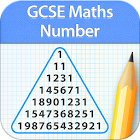GCSE Maths Number Revision LE

All Android applications categories

All Android games categories# GCSE Maths Number Revision LE

78 6.8

6.8 Users
rating

## Screenshots

Description

Take a breath and make your GCSE preparation a fun activity with our collection of GCSE apps.

Here comes the most comprehensive GCSE Maths- Number app.

**NOTE: This is a lite version where in only few topics are available. All the remaining locked topics will be unlocked on buying the full version from within this lite version. It will be a one time purchase to unlock ALL the locked items in one go.

~~~~~~~~~~~~~~~~~~~~~~~~~~~~~~~~~~~~~~~
Full version has 760 questions across 76 subtopics.
~~~~~~~~~~~~~~~~~~~~~~~~~~~~~~~~~~~~~~~~

Features include:

• HIGHEST QUALITY and QUANTITY
760 questions and 76 revision notes in all just for Number!. High quality content written by experienced GCSE tutors.

• REVISE BY TOPIC
Basics, factors, multiplication, division, decimals, fractions, percentages, powers, roots and surds, ratios, speed, proportion and variation, approximation and rounding.

• MOCK TEST
Mixed questions from all topics.

• REVIEW WITH EXPLANATION
Review each question at the end of the test. Know the right answer with detailed explanation for each question.

• PROGRESS METER
With our unique progress tracking feature including pie charts and bar graphs showing your progress, you know you are ready to take on the real test at the board when your progress meter says 100%.

More details on topics:

1. NUMBER BASICS

The number line (F)
Ordering Numbers and Place Values (F)
Negative Numbers (F)
Addition and subtraction with negative numbers (F)
Order of operations and BODMAS (F)
Number problems in words (F)

2. FACTORS, MULTIPLICATIONS AND DIVISION

Times tables (F)
Multiplying and dividing by single-digit numbers (F)
Prime numbers (F)
Prime factors (F)
Factors (F)
Highest common factor (F)
Multiples (F)
Lowest common multiple (F)
Long multiplication (F)
Long division (F)
Multiplying and dividing with negative numbers (F)
Multiplying and dividing by decimals (F)
Real-life problems using multiplication and division (F)

3. FRACTION, DECIMALS AND PERCENTAGES

Fractions of a shape (F)
Finding a fraction of a quantity (F)
Improper fractions and mixed numbers (F)
Equivalent fractions (F)
One quantity as a fraction of another (F)
Converting fractions into decimals (F)
Converting decimals into fractions (F)
Multiplying fractions (F)
Dividing fractions (F)
Reciprocals (F)
Fraction problems in words (F)
Decimals - multiplication and division (F)
Equivalent percentages, fractions and decimals (F)
Calculating a percentage of a quantity (F)
One quantity as a percentage of another (F)
Percentage change (F)
Percentage problems in words (F)
Compound interest (H)
Reverse percentage (H)

4. POWERS, ROOTS AND SURDS

Square numbers (F)
Powers of 10 (F)
Multiplying using powers of 10 (F)
Dividing using powers of 10 (F)
Square roots and cube roots (F)
Multiplying powers (F)
Dividing powers (F)
Calculated Powers (F)
Power of a power (F)
Fractional and zero powers (H)
Negative powers (H)
Standard form (H)
Surds and pi (H)
Rationalising the denominator (H)
Solving problems with surds (H)

5. RATIO, SPEED, PROPORTION AND VARIATION

Dividing amounts in ratios (F)
Ratios and fractions (F)
Calculating with ratios (F)
Ratio problems in words (F)
Speed (F)
Density (H)
Direct proportion (H)
Inverse proportion (H)

6. APPROXIMATION and ROUNDING

Significant figures (F)
Approximation of calculations (F)
Limits of accuracy (F)
Calculating with limits of accuracy (F)
Decimal places (F)
Rounding (F)

from 78 reviews

"Good"

6.8